NAACL 2019

## Intuition

The standard setup for unsupervised structure learning is to define a generative model over observed data (e.g. sentence) and unobserved structure (e.g. parse tree, part-of-speech sequence), and maximize the log marginal likelihood. Classic method to unsupervised parsing have made strong conditional independence assumptions (e.g. context-freeness) and employed auxiliary objectives or priors. This constrain make the likelihood tracable. However, they come at the expense of language modeling performance, particularly compared to sequential neural models that make no independence assumptions.

RNNG make no independence assumptions. That makes RNNG a good language model but also several disadvantages. 1) Marginalization is intractable. 2) The inductive biases imposed by the RNNG are relatively weak compared to those imposed by models like PCFGs.

This work use amortized variational inference with a structured inference network that makes them tractably optimoize a lower bound on the log marginal likelihood, while injecting inductive bias by employing a structured inference network.

## Model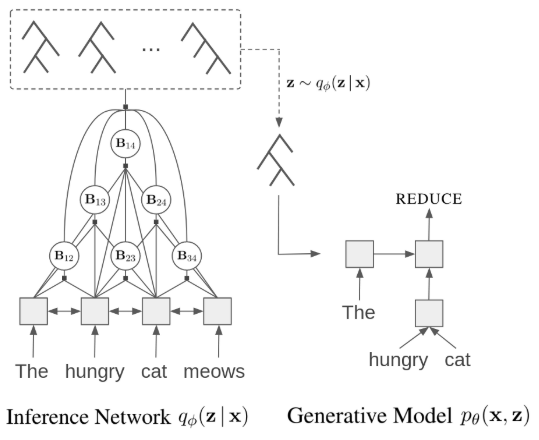Like VAE, there also a infence network (left) and generate network (right). Generate network is a traditonal RNNG generate part. The inference network is a CRF parser.

### Generate objective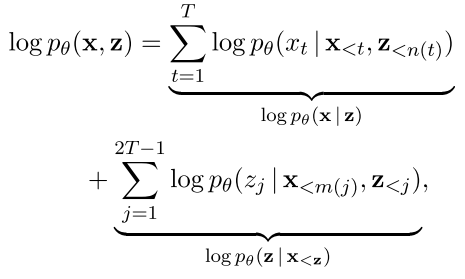In the supervised case where ground-truth z is available, we can straightforwardly perform gradient-based optimization to maximize the joint log likelihood $\log (p_{\theta}(\mathbf{x}, \mathbf{z}))$. In unsupervised case, the standard approach is to maximize the log marginal likelihood: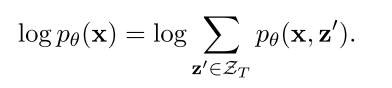But for their model, this summation is intractable.

### Amortized Variational Inference

Here they introduce a inference network $q_{\phi}(\mathbf{z} | \mathbf{x})$, with the inference network, we can optimize an evidience lower nound (ELBO) on the log marginal likelihood: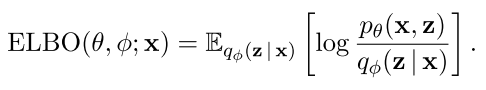We use inference network to calcualte every span score $s_{ij}$ of position from $i$ to $j$. Then calcualte the partition by inside-outside method.

### Optimize

They optimize a variant of the ELBO: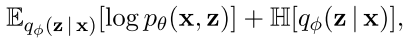The gradient of every part can be calcualte as: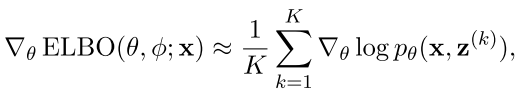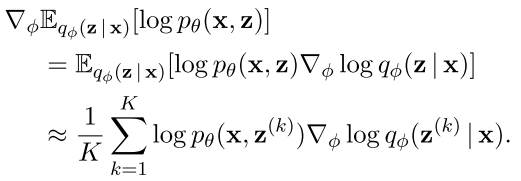For $H$ part, gradient can be calculated by automatic differentation.

### Apendix

Algorithm of samping a tree from inference network: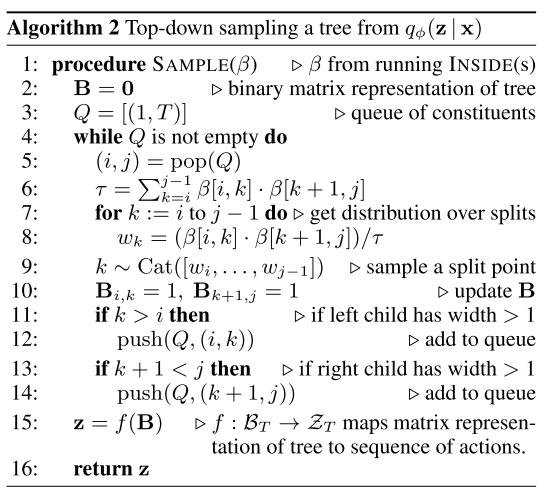Algorithm of tree entropy: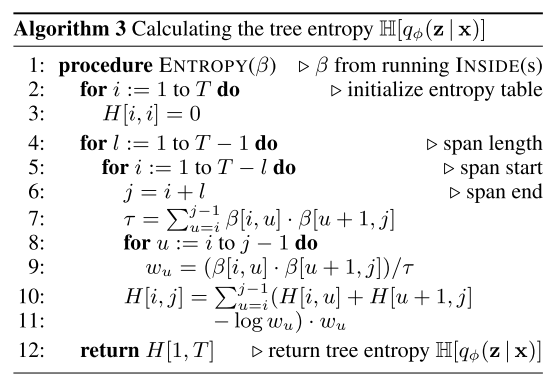TODO read the experiment